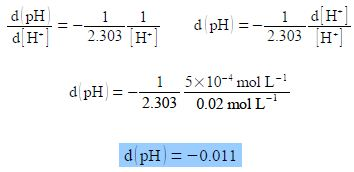# How to Change in pH?

The pH of a solution in which the hydrogen ion concentration is [H] is defined by:

pH = – log[H+]

Firstly, show that d(pH)/d[H+] arising from a small change in pH of d(pH) resulting from a small change in [H+] of d[H+] is proportional to the ratio d[H+]/[ H+]. Secondly, a solution originally with [H+] = 0.02 mol L-1 had an additional concentration of d[H+] = 5x 10-4 mol L-1 added to it, calculate the change in pH, d(pH).

Solution

pH = – log[H+]

As we need to differentiate this, we first need to convert it to natural logarithms. Letting x = [H+]  we have

logb (a) = loga (x) = logb (x)Note the negative sign, we may now differentiate the above equation, then treat the differential as separable terms and then substitute the values for the variables.As expected increasing the hydrogen ion concentration leads to a decrease in pH.